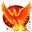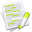#wx.GridSizer¶

A grid sizer is a sizer which lays out its children in a two-dimensional table with all table fields having the same size, i.e.

the width of each field is the width of the widest child, the height of each field is the height of the tallest child.

##Class Hierarchy¶Inheritance diagram for class GridSizer:

wx.FlexGridSizer

##Methods Summary¶

 __init__ wx.GridSizer constructors. CalcMin This method is abstract and has to be overwritten by any derived class. CalcRowsCols CalcRowsCols() . (rows, cols) GetCols Returns the number of columns that has been specified for the sizer. GetEffectiveColsCount Returns the number of columns currently used by the sizer. GetEffectiveRowsCount Returns the number of rows currently used by the sizer. GetHGap Returns the horizontal gap (in pixels) between cells in the sizer. GetRows Returns the number of rows that has been specified for the sizer. GetVGap Returns the vertical gap (in pixels) between the cells in the sizer. RepositionChildren Method which must be overridden in the derived sizer classes. SetCols Sets the number of columns in the sizer. SetHGap Sets the horizontal gap (in pixels) between cells in the sizer. SetRows Sets the number of rows in the sizer. SetVGap Sets the vertical gap (in pixels) between the cells in the sizer.

##Class API¶

class wx.GridSizer(Sizer)

Possible constructors:

GridSizer(cols, vgap, hgap)

GridSizer(cols, gap=Size(0,0))

GridSizer(rows, cols, vgap, hgap)

GridSizer(rows, cols, gap)


A grid sizer is a sizer which lays out its children in a two- dimensional table with all table fields having the same size, i.e.

### Methods¶

__init__(self, *args, **kw)

wx.GridSizer constructors.

Usually only the number of columns in the flex grid sizer needs to be specified using cols argument. The number of rows will be deduced automatically depending on the number of the elements added to the sizer.

If a constructor form with rows parameter is used (and the value of rows argument is not zero, meaning “unspecified”) the sizer will check that no more than cols*rows elements are added to it, i.e. that no more than the given number of rows is used. Adding less than maximally allowed number of items is not an error however.

Finally, it is also possible to specify the number of rows and use 0 for cols. In this case, the sizer will use the given fixed number of rows and as many columns as necessary.

The gap (or vgap and hgap, which correspond to the height and width of the wx.Size object) argument defines the size of the padding between the rows (its vertical component, or vgap) and columns (its horizontal component, or hgap), in pixels.

New in version 2.9.1: (except for the four argument overload)Overloaded Implementations:

__init__ (self, cols, vgap, hgap)

Parameters
• cols (int) –

• vgap (int) –

• hgap (int) –

__init__ (self, cols, gap=Size(0,0))

Parameters

__init__ (self, rows, cols, vgap, hgap)

Parameters
• rows (int) –

• cols (int) –

• vgap (int) –

• hgap (int) –

__init__ (self, rows, cols, gap)

Parameters
• rows (int) –

• cols (int) –

• gap (wx.Size) –

CalcMin(self)

This method is abstract and has to be overwritten by any derived class.

Here, the sizer will do the actual calculation of its children’s minimal sizes.

Return type

wx.Size

CalcRowsCols(self)

Calculates how many rows and columns will be in the sizer based on the current number of items and also the rows, cols specified in the constructor.

GetCols(self)

Returns the number of columns that has been specified for the sizer.

Returns zero if the sizer is automatically adjusting the number of columns depending on number of its children. To get the effective number of columns or rows being currently used, see GetEffectiveColsCount

Return type

int

GetEffectiveColsCount(self)

Returns the number of columns currently used by the sizer.

This will depend on the number of children the sizer has if the sizer is automatically adjusting the number of columns/rows.

Return type

int

New in version 2.9.1.

GetEffectiveRowsCount(self)

Returns the number of rows currently used by the sizer.

This will depend on the number of children the sizer has if the sizer is automatically adjusting the number of columns/rows.

Return type

int

New in version 2.9.1.

GetHGap(self)

Returns the horizontal gap (in pixels) between cells in the sizer.

Return type

int

GetRows(self)

Returns the number of rows that has been specified for the sizer.

Returns zero if the sizer is automatically adjusting the number of rows depending on number of its children. To get the effective number of columns or rows being currently used, see GetEffectiveRowsCount .

Return type

int

GetVGap(self)

Returns the vertical gap (in pixels) between the cells in the sizer.

Return type

int

RepositionChildren(self, minSize)

Method which must be overridden in the derived sizer classes.

The implementation should reposition the children using the current total size available to the sizer ( m_size ) and the size computed by the last call to CalcMin .

Note that you should never call this method directly, call Layout instead if you need to manually update the sizer elements positions. This method is only called by wxWidgets itself.

Parameters

minSize (wx.Size) –

New in version 4.1/wxWidgets-3.1.3: , before this version RecalcSizes() method not taking any arguments had to be overridden in the derived classes instead.

SetCols(self, cols)

Sets the number of columns in the sizer.

Parameters

cols (int) –

SetHGap(self, gap)

Sets the horizontal gap (in pixels) between cells in the sizer.

Parameters

gap (int) –

SetRows(self, rows)

Sets the number of rows in the sizer.

Parameters

rows (int) –

SetVGap(self, gap)

Sets the vertical gap (in pixels) between the cells in the sizer.

Parameters

gap (int) –

### Properties¶

Cols

EffectiveColsCount

EffectiveRowsCount

HGap

Rows

VGap